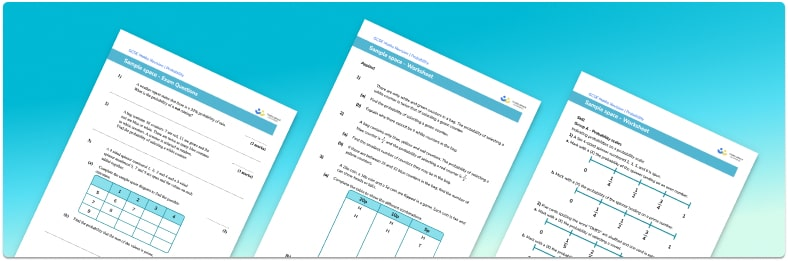# Sample Space Worksheet• Section 1 of the sample space worksheet contains 20+ skills-based sample space questions, in 3 groups to support differentiation
• Section 2 contains 4 applied sample space questions with a mix of worded problems and deeper problem solving questions
• Section 3 contains 3 foundation and higher level GCSE exam style sample space questions
• Answers and a mark scheme for all sample space questions are provided
• Questions follow variation theory with plenty of opportunities for students to work independently at their own level
• All questions created by fully qualified expert secondary maths teachers
• Suitable for GCSE maths revision for AQA, OCR and Edexcel exam boards

• This field is for validation purposes and should be left unchanged.

You can unsubscribe at any time (each email we send will contain an easy way to unsubscribe). To find out more about how we use your data, see our privacy policy.

### Sample space at a glance

Sample space diagrams visually represent all of the possible outcomes of an event, or two separate events. They are useful in helping us systematically list all of the outcomes without making repeats or missing outcomes. Once we have drawn a sample space diagram, we can use them to answer probability questions.

The symbol Ω (the capital greek letter Omega) is used to list items within the sample space. The sample space for a fair spinner could be written as the set Ω = {Red, Yellow, Green, Blue} where the probability of each colour would be 1 out of 4.

We can also draw a sample space diagram that shows the different outcomes when we toss a fair coin and roll a fair die. The outcomes for the coin can go down the side of the table (as rows) with the outcomes for rolling the six sided die across the top (as columns). Each box within the table is filled in with the outcome of the two events for that row and column. The sample space is:

1 2 3 4 5 6

Head H1 H2 H3 H4 H5 H6

Tail T1 T2 T3 T4 T5 T6

Looking at this table, we can see that there are a total of 12 different outcomes (H1, T1, H2, T2, etc). The probability of getting an odd number is  6 out of 12, the probability of getting an even number and a tail is 3 out of 12 and the probability of getting a prime number or a head is 9 out of 12.

Looking forward, students can then progress to additional probability worksheets, for example the Venn diagrams worksheet, the conditional probability worksheet, or the probability tree diagrams worksheet.For more teaching and learning support on probability our GCSE maths lessons provide step by step support for all GCSE maths concepts.

## Do you have KS4 students who need more focused attention to succeed at GCSE?There will be students in your class who require individual attention to help them succeed in their maths GCSEs. In a class of 30, it’s not always easy to provide.

Help your students feel confident with exam-style questions and the strategies they’ll need to answer them correctly with our dedicated GCSE maths revision programme.

Lessons are selected to provide support where each student needs it most, and specially-trained GCSE maths tutors adapt the pitch and pace of each lesson. This ensures a personalised revision programme that raises grades and boosts confidence.

Find out more